# 翁恺 C 语言程序设计网课笔记合集

• 2022 年 8 月 02 日
• 本文字数：44423 字

阅读完需：约 146 分钟[TOC]

# 1. 背景

## 计算机和编程语言

//例：hello world!#include<stdio.h>int main(){  printf("Hello World!\n");//输出“Hello World!（换行）”引号内的部分  return 0;}

## 计算机的思维方式

1. 枚举法代入 x=1，不行；代入 x=2，不行；代入……

2. 二分法代入 x=5，小了；代入 x=7……

1. 解释：借助一个程序，那个程序可以试图理解你的程序，然后按照你的要求执行

2. 编译：借助一个程序把你的程序翻译成机器语言写的程序，然后计算机就可以直接执行了。

## 为什么编程入门从 C 学起？1. 在很多场合，C 语言是唯一的选择

2. 现代编程语言在语法上差异很小，基本都和 C 接近

3. 语言的能力、适用领域主要是由 传统 决定的。

## C 的简单历史

1969 诞生。FORTRAN→BCPL→B 语言→C 语言

1967 年，剑桥大学的 Martin Richards 对 CPL 语言进行了简化，于是产生了 BCPL（Basic Combined Pogramming Language)语言。1970 年，美国贝尔实验室的 Ken Thompson。以 BCPL 语言为基础，设计出很简单且很接近硬件的 B 语言（取 BCPL 的首字母）。并且他用 B 语言写了第一个 UNIX 操作系统。在 1972 年，美国贝尔实验室的 D.M.Ritchie 在 B 语言的基础上最终设计出了 C 语言。因为 B 和 BCPL 都支持指针间接方式，所以 C 语言也支持了。C 语言还受到了 PL/I（和底层系统很接近，可以拿来做操作系统等）的影响，还和 PDP-II 的机器语言有很大关系 1973/3，第三版的 Unix 上出现了 C 语言的编译器 1973/11，第四版的 Unix 完全用 C 语言重新写的。## 编程软件用什么

C 语言的用途：写操作系统、嵌入式系统、驱动程序（用来驱动 U 盘）、底层驱动、图形引擎、图像处理、声音效果（直接给我们提供数据的代码不是用 C 语言写的）一些底层的东西。C 语言是一种工业语言，由基础人员使用。所以：开发效率>>学习过程开发效率>>开发乐趣而在日常的应用中，很少直接用 C 语言编写。学习 C 的过程主要是写练习代码，而不是写真实软件。C 语言需要被编译才能运行，所以需要：编辑器和编译器（或者 IDE，Integrated Development Environment 集成开发环境，一个顶俩）推荐的编程软件：Dev C++。免费，安装简单，还不用建工程。# 2. 入门：从 Hello World 开始

## 第一个 C 程序

#include<stdio.h>int main(){  printf("Hello World!\n");  return 0;}

## 详解第一个 C 程序

#include<stdio.h>int main(){    return 0;}

## 简单计算

printf("%d",23+43);

%d 是占位符，说明后面会有一个整数输出到这个位置上。

printf("23+43=%d",23+43);

# 3. 变量

## 变量定义

1. 有办法输入数字；

2. 有地方放输入的数字；

3. 输入的数字参与计算。

  int price=0;//定义了整形变量price，类型是int，初始值=0  printf("请输入金额（元）：");  int change=100-price;  printf("找您%d元。\n",change);

## 变量赋值与初始化

  int price=0;//其中=是赋值运算符，把右边的值赋给左边的变量。

  int a=0,b=1;

//C语言  int price=0;  printf("请输入金额（元）：");  scanf("%d",&price);  int change=100-price;  printf("找您%d元。\n",change);

//传统的ANSI C只能在开头的地方定义变量  int price=0;  int change=0;    printf("请输入金额（元）：");  scanf("%d",&price);  change=100-price;  printf("找您%d元。\n",change);

## 变量输入

scanf()函数，读到的结果赋值给后面的变量（注意变量前的 &）如果输入非整数（比如找钱一例中：输入字母，找我 100 元）应该怎么处理？这种情况后面再说。

## 常量 vs 变量

  const int amount=100;

## 浮点数

C 语言中两个整数做运算得到的结果也只能是个整数（去掉小数部分）。而 10 和 10.0 在 c 中是完全不同的两个数字，10.0 是浮点数（浮点数指小数点时可以浮动的，是计算机中表示分数和无理数的一种方式。人们用浮点数来称呼有小数点的数）（其实还有定点数。但是在 c 语言中无）当浮点数和整数放在一起运算时，计算机会自动把整数转化为浮点数计算（如：

int a,b;printf("a/b*3");//改进为a/b*3.0

## 表达式int hour1,min1;  int hour2,min2;    scanf("%d %d",&hour1,&min1);  scanf("%d %d",&hour2,&min2);    int t1=hour1*60+min1;//把小时转化为分钟单位  int t2=hour2*60+min2;    int t=t2-t1;  printf("时间差是%d小时%d分。“,t/60,t%60);//t/60是小时部分；t%60取余，是分钟部分

## 运算符优先级

（double 输入的时候用%lf，输出的时候用%f就行）运算符优先级

  result=a=b=3+c;  result=(result=result*2)*6*(result=3+result);

## 交换变量

a=b;b=a;

c=a;a=b;b=c;DEV C++中，在左边数字那里点击一下，然后程序运行时就会在这一行停止。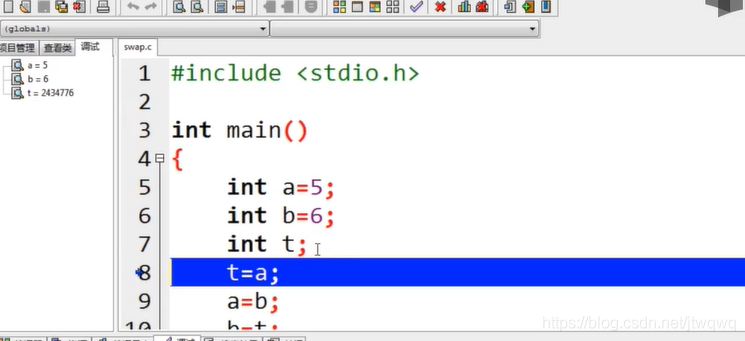## 复合赋值

a+=5表示a=a+5(注意两个运算符之间无空格)类似有-=*=/=a*=b+5表示a=a*(b+5),如果想先算a=a*b记得加括号）INC 递增、DEC 递减运算符：++--：单目运算符，只能有一个算子，这个算子还只能是变量（如a++后缀形式，++a前缀形式）让该变量+1/-1；a++运算式的值是 a+1 以前的值；++a运算式的值是 a+1 以后的值。无论哪个，a 的值都+1 了++--可以单独使用，但不要把它组合进表达式，复杂。

# 4. 判断（选择结构）

if 条件判断

  if(条件){    要执行的语句，只有条件成立时才会执行  }

## 判断的条件

printf("%d\n",5>3);printf("%d\n",5==3);

## 找零计算器

st=>start: 开始i1=>inputoutput: 输入购买的金额i2=>inputoutput: 输入支付的票面o1=>operation: 计算找零c=>condition: 判断余额是否充足o2=>inputoutput: 打印找零o3=>inputoutput: 告知用户余额不足以购买e=>end: 结束st->i1->i2->o1->cc(yes)->o2->ec(no)->o3->e

  //初始化  int price=0;  int bill=0;  //读入金额和票面  printf("请输入金额：");  scanf("%d",&price);  printf("请输入票面：");  scanf("%d",&bill);  //计算找零

//是（单行）注释，把程序分成了三个部分：初始化，读入金额和票面，计算并打印找零；c99 可以，ASCII 不支持 comment 对程序的功能没有任何影响，但是往往能使程序更容易被人类读者理解。/* */中间可以有多行注释。

## 否则

  int a,b;  printf("请输入两个整数：");  scanf("%d %d",&a,&b);  int max=0;  if(a>b)max=a;  else max=b;  printf("大的那个是%d\n",max);

  int a,b;  printf("请输入两个整数：");  scanf("%d %d",&a,&b);  int max=b;  if(a>b)max=a;  printf("大的那个是%d\n",max);

## 没有大括号的 if 语句

if 语句可以不加大括号

if（a>b）  max=a;

If 这行结束并没有语句结束的标志;而后面的赋值语句写在下一行且缩进了，结束时有“；”。这表明这条赋值语句是 if 语句的一部分，if 语句拥有和控制这条赋值语句，决定他是否要被执行。就是说，没有大括号，后面一句话是有效的，也只能这一句是有效的（包含在 if 里）。

## 嵌套的 if-else

if(a>b){  if(a>c)max=a;  else max=c;}else{  if(b>c)max=b;  else max=c;}

## 级联的 if-else if

flowchatst=>start: 开始i=>inputoutput: 输入o=>inputoutput: 输出c1=>condition: 是否满足条件1c2=>condition: 是否满足条件2o1=>inputoutput: 输出条件1o2=>inputoutput: 输出条件2o3=>inputoutput: 输出条件3en=>end: 结束st->i->c1c1(yes)->o1->enc1(no)->c2c2(yes)->o2->enc2(no)->o3->en

else{  if()  else}

if(x<0)f=-1;else if(x==0)f=0;else f=1;printf("%d",f);

if(x<0)printf("%d",-1);else if(x==0)printf("%d",0);else printf("%d",1);

## if-else 的常见错误

1. 忘记写大括号的话，条件成立时只执行后面一句

2. If 后面无分号

3. ==和=：if 只要求括号里是 0 或非 0

4. 使人困惑的 else 尊重 warning！大括号内的语句加 tab 缩进是代码风格。3.2.4 多路分支

switch(print){  case 1:    printf("1");    break;  case 2:    printf("2");    break;  case 3:    printf("3");    break;  default:    printf("0");}

int i=1;switch ( i%3 ) {case 0: printf("zero");case 1: printf("one");case 2: printf("two");}

# 5. 循环（循环结构）

if(n!=0){  n/=10;i++;}if(n!=0){  n/=10;i++;}

……不过这样也是无限循环。所以我们可以引入新函数，while。条件满足，就会不断重复大括号里的内容。（尝试的时候不要拿太大的数去试，计算机里的整数是有范围的。）

## while 循环

1. 用户输入 x；

2. 初始化 n 为 0；

3. X/=10，去掉个位；

4. cnt++;

5. 如果 x>0，回到 3；

6. 否则 cnt 就是结果。

do-while 循环：进入循环的时候不做检查，而是执行完一轮循环体的代码之后再来检查循环条件是否满足，满足的话继续，不满足的话结束循环(while();最后一定要有分号！！！)while 和 do while 很像，区别在于 do while 在循环体执行结束时才来判断条件。也就是说无论如何，循环都会执行至少一遍。

## do-while 循环

if 和 while 的区别在于，if 判断只一次，不管结果如何都结束了。**While 一定要有结束循环的条件！！**否则会一直循环，超时 While 可以翻译为当。循环有可能一次都没有被执行。条件成立是循环成立的条件。如何看出程序运行结果？人脑模拟计算机的运行，在纸上列出所有变量，随着程序的进展不断重新计算变量的值。当程序运行结束时，留在表格最下面的就是程序的最终结果。测试程序常常使用边界数据，如有效范围两端的数据、特殊的倍数等等。（此题求位数，特殊数据可以是个位数、10、0、负数）然后可以发现，0 是 1 位数，但是用 while 算法的话算出来是 0 位数。那我们可以用 do-while 就能得到 1 位数了。或者 if(x>0),做 while；else 位数=1，单独列出 x=0 的情况。另一种调试方法：在适当的位置加上 printf 输出作用不只有输出数据。比如在 while 括号内加一个 printf(“in loop”);证明程序到这个地方了，也就是进入 while 循环了（还可以看循环了几次）

## 循环计算

• While 可以用 do while 吗？

• 为什么计数从 0 开始，可以从 1 开始吗？

• 为什么 while 判断条件是 x>1?

• 循环最后输出的是多少？

#include<stdio.h>int main(){  int x,ret=0;  scanf("%d",&x);  int t=x;  while(x>1){    x/=2;    ret++;  }  printf("log2 of %d is %d.",t,ret);  return 0;}

## 猜数游戏

1. 因为要不断重复去猜，所以我们要用到循环

2. 实际写出程序之前，我们可以先用文字描述出程序的思路。

3. 核心重点是循环的条件。

4. 人们往往会考虑循环终止的条件。

st=>start: 开始i=>inputoutput: 用户输入猜的数ocnt=>operation: count++o=>operation: 计算机随机想一个数，记在number里c=>condition: 判断a是否等于numbere=>end: 结束o2=>inputoutput: 告诉用户大了还是小了oe=>operation: 输出cnt猜的次数st->o->i->ocnt->cc(yes)->oe->ec(no)->o2(right)->i

//先加入两个头文件#include<stdlib.h>和#include<time.h>#include<stdio.h>#include<stdlib.h>#include<time.h>int main(){  srand(time(0));//Main里加上srand(time(0));  先不用管什么意思  int a=rand();//如果想要a是100以内的整数：用取余即可（a%=100）  int number=rand()%100+1;//这样召唤出来的数范围是1~100  //不管怎样用户都要进入这个循环，输入至少一个数；所以应该用do-while循环。}

## 算平均数

flowchatst=>start: sum=0,cnt=0i1=>inputoutput: 读numc=>condition: num!=-1?o=>operation: sum+=num, cnt++op=>inputoutput: 计算和打印结果e=>end: endst->i1->cc(yes)->o->i1c(no,left)->op->e

## 循环控制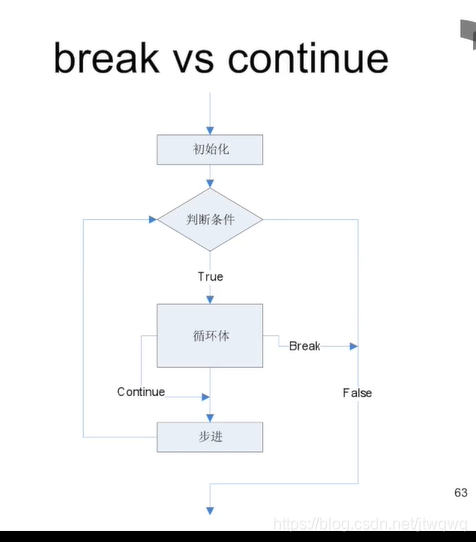## 嵌套的循环

int x;//再scan xint isprime=1;for(int i=2;i<x;i++){  if(x%i==0){    isprime=0;    break;  }}//再根据isprime是不是1判断x是不是素数

## 从嵌套的循环中跳出

for(one=1;one<x*10;one++){  for(two=1;two<x*10/2;two++)  {    for(five=1;five<x*10/5;five++)//break跳出的是这层循环，而没有跳出上面的两层循环    {      if(one+two*2+five*5==x*10)      {        printf("可以用%d个一角加%d个两角加%d个五角得到%d元\n",one,two,five,x);        break;      }    }  }}

//接力breakint x,one,two,five,x;int exit=0;scanf("%d",&x);for(one=1;one<x*10;one++){  for(two=1;two<x*10/2;two++)  {    for(five=1;five<x*10/5;five++)    {      if(one+two*2+five*5==x*10)      {        printf("可以用%d个一角加%d个两角加%d个五角得到%d元\n",one,two,five,x);        exit=1;        break;      }    }    if(exit==1)break;  }  if(exit==1)break;}

//gotoint x,one,two,five,x;int exit=0;scanf("%d",&x);for(one=1;one<x*10;one++){  for(two=1;two<x*10/2;two++)  {    for(five=1;five<x*10/5;five++)    {      if(one+two*2+five*5==x*10)      {        printf("可以用%d个一角加%d个两角加%d个五角得到%d元\n",one,two,five,x);        goto out;      }    }  }}out:return 0;

（除了这种多重 break 的结构建议使用 goto，别的地方不建议。）

# 补充：PTA 例题

## 前 n 项和

#include<stdio.h>int main(){  int n;  double sum=0.0;  scanf("%d",&n);  for(int i=1;i<=n;i++)  {    sum+=1.0/i;  }  printf("f(%d)=%f",n,sum);  return 0;}

sum+=sign*1.0/i;sign=-sign;

1. 先求 x 的逆序数 t

2. 逆序逐位输出 t

## 求最大公约数

循环t++if(u/t==0&&v/t==0)gcd=t;//不断刷新gcdif(t==u||t==v)break;//并输出gcd;

1. 如果 b=0，计算结束，a 就是最大公约数；

2. 否则，让 a=b，b=a%b；

3. 回到第一步（可以使用之前提到过的变量表格法来人工运行）

## 求符合给定条件的整数集

int i,a,j,k,cnt=0;    scanf("%d",&a);    i=a;    //思路：i,j,k都从a开始，从小到大逐渐增加到a+3，三者不能相等        while(i<=a+3){        j=a;        while(j<=a+3){            k=a;            while(k<=a+3){               if(i!=j&&j!=k&&i!=k){                   printf("%d%d%d",i,j,k);                   cnt++;                   if(cnt==6){                       printf("\n");                       cnt=0;                   }                   else printf(" ");               }               k++;            }            j++;        }        i++;    }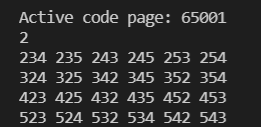## 打印乘法口诀表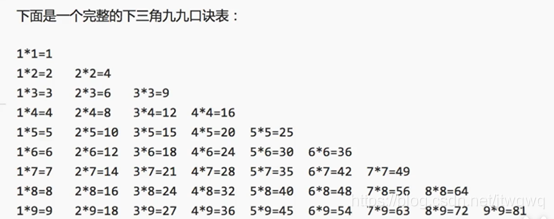a*b，a、b 两重循环。还要注意对齐问题。如果结果是一位数，输出两个空格；两位数输出一个空格。

## n 项求和

2/1+3/2+5/3+8/5+……的前 n 项之和（从第二项开始，每一项的分子是前一项的分子和分母之和，分母是前一项的分子）

dividend=2;//分母divisor=1;//分子for(i=1;i<=n;i++){  sum+=dividend/divisor;  t=dividend;  dividend+=divisor;  divisor=t;}printf("%.2f/n",sum);

## 约分最简分式

1. scanf("%d/%d")这样处理输入

2. 辗转相除法寻找最大公约数；念数字：比如输入-12：fu yi er 先判断-的情况之后 switch case 很好办。以后学会数组之后会有更优解。

# 6. 数据类型

C 语言是有类型的语言。C 语言的变量，必须：1.在使用前定义;2.确定类型.如果我们一开始直接说 a=6:使用了未定义的标识符 a。

C 语言之后语言朝着两个方向发展：

1. C++和 Java 更强调类型，对类型的检查更加严格

2. JavaScript、Python、PHP 不着重类型，甚至不需要事先定义。支持强类型的观点认为明确的类型有助于尽早发现程序中的简单错误；反对强类型的观点认为过于强调类型，迫使程序员面对底层，实现而非事务逻辑

1. 类型名称：int,long,double……

2. 输入输出时的格式化（占位符）：%d,%ld,%lf……

3. 所表达的数的范围：char<short<int<float<double

4. 内存中占据的大小：1~16 个字节

## 整数类型

sizeof(char)=1;//char1字节（8比特）sizeof(short)=2;//short2字节sizeof(int)=4;//由编译器决定，通常代表“1个字”sizeof(long)=4;//由编译器决定，通常代表“1个字”sizeof(long long)=8;//long long 8字节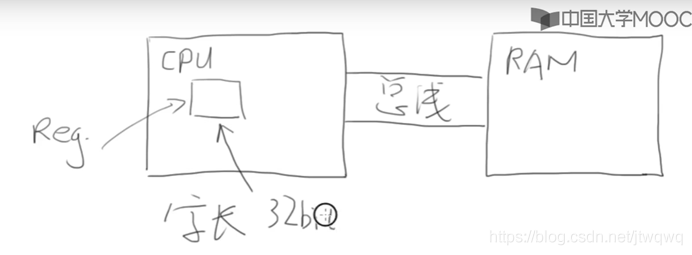## 整数的内部表达

1. 像十进制一样，有一种特殊的标志（类似符号）来表示负数（缺陷：计算机做加减法的时候，要像判断十进制的负号一样，我们需要一个东西去控制加号还是减号。不过每次计算机都要特别地去判断这个符号的正负，这样就会比较复杂。）

2. 从 0000 0000 到 1111 11111，取中间的数为 0，如 1000 0000 表示 0，比他小的是负数，比他大的是正数，各一半（缺陷：所有数都要和这个数做减法来计算其值，会比较复杂）

3. 补码思路：本质上来看，（互为相反数的）负数+正数=0。这是提供思路的一种方法。比如我们希望-1+1→0，如何能够做到？如 0→0000 0000，1→0000 0001，我们让一个数+1 得到 0。这个数字选谁？全 1 的数字 1111 1111。因为 0000 0001+1111 1111→1 0000 0000 多出来的一位（溢出）那一位被丢掉了，相加结果即是 00000000。妙啊或者换个角度：-1=0-1=(1)0000 0000-0000 0001→1111 1111（1111 1111 被当作纯二进制看待时是 255，被当做补码看待时是-1）所以对于-a 来说，他的补码就是 0-a，实际就是 2^n^-a，n 是该种类型的位数补码的意义就是拿补码和原码可以加出一个溢出的 0。另一个好处是这样我们做计算的时候，不需要调整加减，全部都是加法（+补码就是-原码）。

## 整数的范围：如何推算整数类型所能表达的数的范围，越界了会怎样？

char c=255;int i=255;printf("%d%d",c,i);

unsigned char c=255;

unsigned 使得这个类型在正整数表达部分范围扩大一倍，但是不能表达负数了。如果一个字面量常数想要表达自己是 unsigned，可以在后面加 u/U：255USimilarly,想表达自己是个 long,后面加lunsigned 的初衷不是为了扩展数能表达的范围，而是为了做纯二进制运算，主要是为了移位。整数是以纯二进制方式进行运算的。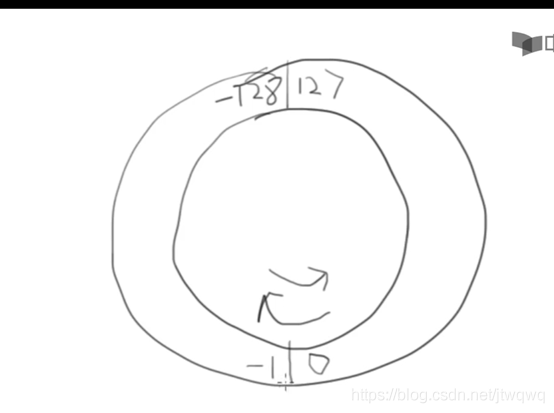char c=127;c++;

unsigned char c=255;c++;

c=0;c--;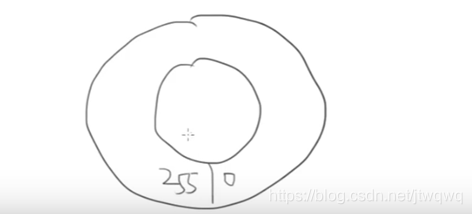int a=0,b=1;while(++a>0);printf("int数据类型的最大数是：%d\n",a-1);while((a/=10)!=0)b++;printf("int数据类型最大的数的数位是:%d",b);

## 整数的格式化：如何格式化地输入输出整数，如何处理 8 进制/16 进制

 char c=012; int i=0x12; printf("c=%d,i=%d\n",c,i);

## 整数类型的选择

char,short,int,long,long long每种类型有不同大小，不同范围，内部是什么样，表达出来是什么样，……C 语言这些又多又复杂的原因：1.C 语言的严谨性，为了准确表达内存，做底层程序的需要 2.C 语言要和硬件打交道，要准确表达计算机里的东西（内存、寄存器、接口……）建议：没有特殊需要，就选择 int 原因 ：

1. 现在 CPU 字长（CPU 和内存之间的通道。如果 char 可能会从 32 位中先挑出来 8 个，可能会花费时间）普遍是 32 位/64 位，一次内存读写、一次计算都是 int，选择更短的类型不会更快，甚至可能会更慢

2. 现代编译器一般会设计内存对齐，（比如这个东西虽然占据 8bit，在内存中仍然占据了一个 int。这个事等到结构再讲）所以更短的类型实际在内存中也可能占据一个 int 的大小。除非在做顶层的硬件，告诉你硬件多大你就要用多大去运算至于 Unsigned 与否，只是输出的形式不同，内部的计算还是一样的

## 浮点类型

float，double32 位，64 位 10^38^,10^308^7 位有效数字，15 但是 float 靠近 0 的部分（0~10^38^）有很小一部分不能表达（这应该是之前的题目为什么 if 判断浮点数不写做差==0，而是写做差<1e-6）float 还能表达 0，+-inf（无穷），nan（非有效数字）%e/%E:科学计数法 1234.56789 输出得到：1.234567e+03-5.67E+16，科学计数法可选的有：+-号，e 或 E，小数点，E 后面的符号+-Double ff=1E-10；输出精度：%.数字f可以指定输出小数点后几位，这样输出的结果是做四舍五入的 %.3f,-0.0045→-0.004%.30f→后面输出 30 位，-0.0049→-0.00489999……（计算机最终只能用离散的数字来表示数字，这就是浮点数的误差。Double 更准确，但可能依然不精确）%.3f，-0.00049→-0.000

## 浮点数的范围和精度

Inf 无穷，nan 输出不存在的浮点数正数除 0.0→inf 负数除 0.0→-inf0.0/0.0→nan(如果是整数，12/0 编译不过)浮点数范围不包括无穷大，但它可以表示。

float a,b,c;a=1.345f;b=1.123f;c=a+b;if(c==2.468)printf("相等");else printf("不相等，c=%.10f，或%f",c,c);

Android计算器低级错误？都是二进制惹的祸！而传统计算器直接用整数做运算而浮点数内部是编码形式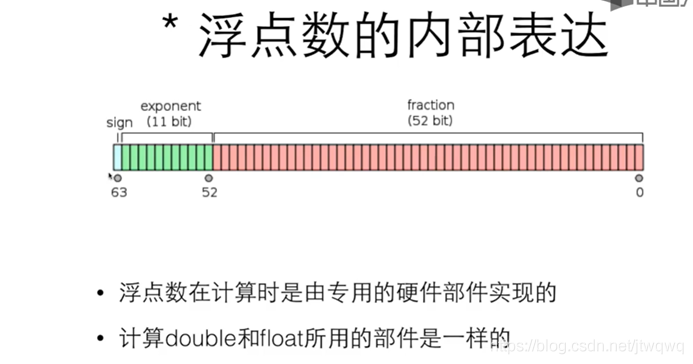## 字符类型：char 是整数也是字符

char 是整数，也是字符。（character）原因 1：我们可以用单引号表示字符的字面量'a','1'''也是字符我们可以在 printf 和 scanf 里用 %c 来输入和输出字符。计算机内部每一个值都有一个字符去表达它（ASCII）如 1 对应的码是 49 输入 1 给计算机的方法：scanf("%c",&c);输入 1 或者scanf("%d",&c);输入 49 输出同样，因为49=='1'注意空格的问题。

scanf("%d%c",&i,&c);scanf("%d %c",&i,&c);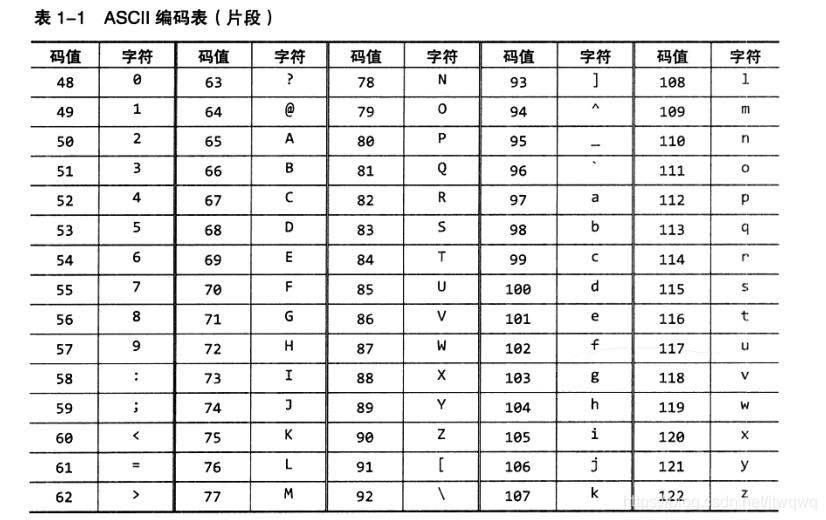## 逃逸字符

printf("请分别输入身高的英尺和英寸，""如输入\"5 7\"表示5英尺7英寸：");

（作业时就会有最后结尾多个空格，使用/b 来删掉的情况，但其实应该说是覆盖了吧，总之这样运行的时候是不正确的，还是要想办法控制输出的空格等的格式正确）例子

printf("123\b\n456\n");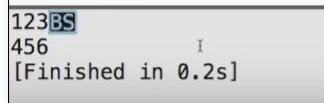printf("123\t456\n");printf("12\t456\n");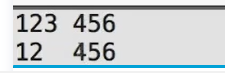\n 换行\r 回车，源自打字机的动作一直敲键盘，打字机的轴就往左移敲一下回车就回到右边，这叫回车然后往上调一下纸，这叫换行。不过 dev c++把回车就当做回车+换行了。

## 类型转换

(int)10.2;(short)32;

int i=32768;short s=(short)i;printf("%d\n",i);

i 输出仍然是 32768。强制类型转换不会改变这个变量自身的类型或值。

double a=1.0;double b=2.0;int i=(int)a/b;

int i=(int)(a/b);(double)(a/b);

## 逻辑类型：表示关系运算和逻辑运算结果的量

bool 类型首先要包含头文件 #include<stdbool.h>，然后可以使用 bool，true,false。

bool b=6>5;

## 逻辑运算：对逻辑量进行与、或、非运算

int a=-1;if(a>0&&a++>1){  printf("OK\n");}printf("%d\n",a);

## 条件运算符

cnt=(cnt>20)?cnt-10:cnt+10;//格式：条件?条件满足时的值:条件不满足时的值

if(cnt>20)cnt-=10;else cnt+=10;

i=3+4,5+6;//编译时会提示warning。因为赋值的优先级也要高于逗号，该式子其实是(i=3+4),(5+6);//右边编译错误,i=7如果写作i=(3+4,5+6);//编译时同样会warning，因为3+4没有用到;i=11

for(i=0,j=10;i<j; i++,j--)

# 7. 函数

## 初见函数

int isPrime(int i){  int ret=1;  int k;  for(k=2;k<i-1;k++){    if(i%k==0){      ret=0;      break;//有1~i-1间的因数就可以判断i不是素数了，break节约时间    }  }  return ret;}

//闭区间内所有数求和的函数 void sum(int begin,int end) {   int i,sum=0;   for(i=begin;i<=end;i++){     sum+=i;   }   printf("%d到%d的和是%d\n",begin,end,sum); }int main(){  sum(1,10);  return 0;}

## 从函数中返回

int函数会有返回值returnreturn停止函数的执行，并返回一个值return;return 一个值;可以写 c=函数()；这样 c=函数的返回值。可以把这个值赋给变量/传给函数/丢掉。没有返回值的函数:void，不能使用带值的return ，可以没有return 。调用的时候也不可以做返回值的赋值。

## 函数原型：用来告诉编译器这个函数长什么样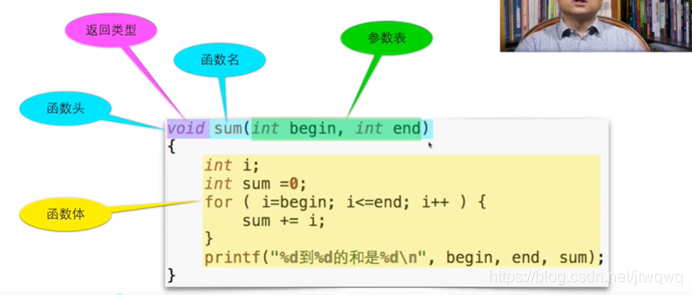void sum(int begin,int end);//声明int main(){  //略去不表，其中用到了sum(a,b)}void sum(int begin,int end)//定义{  int i,sum=0;   for(i=begin;i<=end;i++){     sum+=i;   }   printf("%d到%d的和是%d\n",begin,end,sum);}

double max(double a,double b);

Void sum(int ,int)

## 参数传递：调用哪个函数的时候，是用表达式的值来初始化函数的参数

  void swap(int a,int b)//形参int main(){  int a=5;  b=6;  swap(a,b);//实参} void swap(int a,int b)//形参 {   int t=a;   a=b;   b=t; }

## 本地变量：定义在函数内部的变量是本地变量，参数也是本地变量

### 变量的生存期和作用域

  void swap(int a,int b)//形参int main(){  int a=5;  b=6;  swap(a,b);//实参} void swap(int x,int y)//形参 {   int t=x;   x=y;   y=t; }

1. 本地变量定义在块内。可以是函数的块内，语句的块内，如：if (a<b)int i=10;离开 if 语句之后未定义使用 i 编译错误。（没声明）程序运行进入这个块前，其中的变量不存在。离开后就消失了。如果在块里定义了块外已经定义过的变量，就把块外的变量掩盖了；出来之后又回到块外的值。（C 语言）但是不能在块里面定义同名变量（多次定义）本地变量不会被默认初始化，不会得到一个初始值。而参数进入函数的时候被初始化了。

# 8. 数组

## 初试数组

int number;//定义数组，表示数组可以放100个intscanf(“%d”,&x);while(x!=-1){  number[cnt]=x;//对数组中的元素赋值  cnt++;  scanf(“%d”,&x);}

if(cnt>0){  int i;  double average=sum/cnt;  for(i=0;i<cnt;i++){    if(number[i]>average){//使用数组中的元素      printf("%d ",number[i]);//遍历数组    }  }}

## 数组的使用：如何定义和使用数组，数组的下标和下标的范围

int grades;double weight;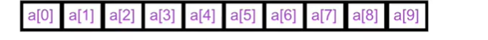## 数组的例子：统计个数

for(int i=0;i<10;i++)count[i]=0;

for(int i=0;i<10;i++)printf(“%d\n”,count[i]);

1. 确定数组大小；

2. 定义数组；

3. 初始化数组；

4. 数组参与运算；

5. 遍历数组输出。

## 数组运算

int a[]={2,4,6,7,1,3,5,9}/*直接用大括号给出数组所有元素的初始值；不需要给出数组的大小，编译器替你数了。*/

int a={=2,=3,6};这个例子里，a=2，a=3，a=6 我们也可以不给出数组大小，让编译器计算。比如上例可写为：int a[]={=2,=3,6};这样会根据大括号里最大的数字下标来算数组的大小。即下标最大为 3 这样特别适合初始数据稀疏的数组。

## 数组的大小

sizeof 给出整个数组所占据的内容的大小，单位是字节。（n*4，sizeof(a)/sizeof(a)就能得到数组元素个数）不能直接把一个数组赋给另一个数组b[]=a;==数组变量本身不能被赋值==。如果想把一个数组的值全部交给另一个数组，必须遍历。

## 数组例子：素数

for(int i=3;i<x;i+=2)

for(int i=3;i<=sqrt(x);i+=2)

int isPrime(int x,int knowsPrimes[],int numberofKnownPrimes)int main(){  const int number=100;  int prime[number]={2};  int count=1;  int i=3;  while(count<number){    if(isPrime(i,prime,count)){      prime[count++]=i;    }    i++;  }//prime数组装着所有素数  for(i=0;i<number;i++){    printf("%d",prime[i]);    if((i+1)%5)printf("\t");    else printf("\n");  }  return 0;} int isPrime(int x,int knowsPrimes[],int numberofKnownPrimes) {   int ret=1;   int i;   for(i=0;i<numberofKnownPrimes;i++){     if(x%knownPrimes[i]==0){       ret=0;       break;     }   }   return ret; }

while(count<number){  if(isPrime(i,prime,count)){    prime[count++]=i;  }  {    printf("i=%d \tcnt=%d\t",i,count);    int i;    for(i=0;i<number;i++){      printf("%d\t",prime[i]);    }    printf("\n");  }  i++;}

{  int i;  printf("\t\t\t\t");  for(i=0;i<number;i++){    printf("%d\t",i);  }  printf("\n");}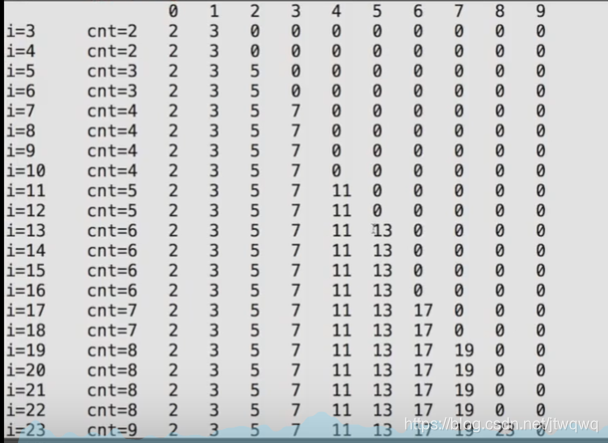1. 令 x=2

2. 将 2x,3x……直到 ax<n 的数标记为非素数

3. 令 x 为下一个没有被标记为非素数的数，重复 2；直到所有的数都已经被尝试完毕。（从 2,3,4,5,6,7,8……删掉 2 的所有倍数，再删掉 3 的所有倍数，再删掉 5 的所有倍数……）

4. 先开辟数组 prime[n]，初始化其所有元素为 1，prime[x]=1 表示 x 是素数，prime[x]=0 表示 x 不是素数

5. 令 x=2

6. 如果 x 是素数，则for(int i=2;x*i<n;i++)prime[i*x]=0

7. x++，重复 3 直到 x==n，否则结束。

int main(){  const int maxNumber=25;  int isPrime[maxNumber];  int i,x;  for(i=0;i<maxNumber;i++)  {    isPrime[i]=1;  }  for(x=2;x<maxNumber;i++)  {    if(isPrime[x])    {      for(i=2;i*x<maxNumber;i++) isPrime[i*x]=0;    }  }  printf("\n");  return 0;}

## 二维数组

int a;通常理解为 a 是一个 3 行 5 列的矩阵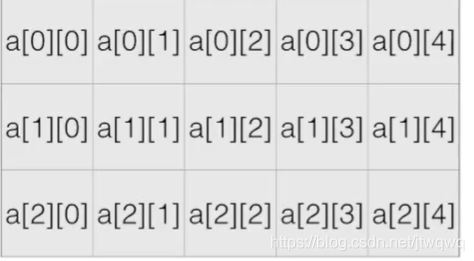int a[]={  {0,1,2,3,4},  {2,3,4,5,6},};

a[i][j]表示一个 inta[i,j]是逗号运算符（等于逗号右边的值），表示a[j]，不是正确表达二维数组的方式。二维数组的列数必须给出，行数可以交给编译器来数。

const int size = 3;int board[size][size];int i,j;int num0fX;//X是一方int num0fO;//O是一方int result=-1;//-1：平局，1：X方赢，0:O方赢//读入矩阵for(i=0;i<size;i++){  for(j=0;j<size;j++){    scanf("%d",&board[i][j]);  }}//检查行for(i=0;i<size&&result==-1;i++){  num0fO=num0fX=0;  for(j=0;j<size;j++){    if(board[i][j]==1)num0fX++;    else num0fO++;  }  if(num0fO==size)result=0;//O方赢  else if(num0fX==size)result=1;//X方赢}

# 9. 指针

## 取地址运算：&运算符取得变量的地址

scanf 里一定要加 &（运算符）作用：取得变量的地址，其操作数必须是变量 C 语言的变量是放在内存里的，&是取出该变量的地址

printf("0x%x",&i);//%x是以16进制输出；&i是一个地址printf("%p",&i);p=(int)&i;printf("0x%x",p);

int a;int b;

## 指针 就是记录地址的变量

int i;int *p=&i;//（p指向了i）*p理解为指向p指针地址所存在的值=i的地址。int* p,q;int  *p,q;

void f(int*p);int main(){  int i=6;  printf("&i=%p\n",&i);  f(&i);  return 0;}void f(int *p){  printf("p=%p\n",p);}//该函数在被调用的时候得到了某个变量的地址

void f(int *p);

p 是地址，*p 是该地址中的数。

//我们调用的时候可以这么写：int i=0;f(&i);

## 指针的用处

1. 现在在函数里利用指针就能使交换两个变量成为可能。可以直接对该地址的变量改变值。和之前的 swap 函数（传递的是两个值）不一样。

void swap(int *pa,int *pb){  int t=*pa;  *pa=*pb;  *pb=t;}

写一个求最大值最小值的函数，要返回最大值和最小值两个值。int a,min,max;minmax(a,sizeof(a)/sizeof(a),&min,&max);void minmax(int a[],int len,int *min,int *max)/*sizeof(a)/sizeof(a)是数组元素个数。函数内部：一个len次的循环来比较每一个a[i]和*min,*max大小，然后令*min,*max不断改变主函数中min和max的值

1. 函数要返回运算的状态，结果通过指针返回。

给你两个数做除法，当分母等于0时返回0，分母不等于0的时候要返回1和除法的结果。int divide(int a,int b,int *result){  int ret=1;  if(b==0)ret=0;  else{    *result=a/b;  }  return ret;}如果返回1再输出a/b结果。

## 指针与数组：为什么数组函数之后的 sizeof 不对了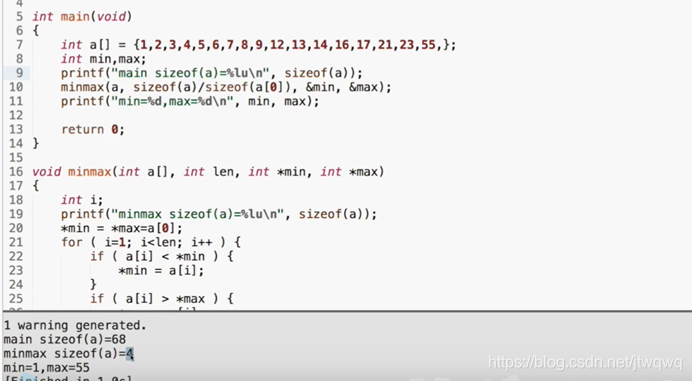sizeof(a)（a 是个数组）返回的是 int*的 sizeof，而不是 int []的 sizeof 如果我们在 minmax 函数中改变 a的值，在 main()函数中 a的值也会被改变，和指针一样。可以看出函数参数表中 int a[]这其实是个指针！（在函数的注释中可以写int a[]，也可以写*a）但我们仍然可以用数组的运算符[]进行运算所以下面这四种函数原型是等价的

int sum(int *ar,int n);int sum(int *,int);int sun(int ar[],int n);int sum(int [],int);

pritnf("min=%d,max=%d\n",min,max);int *p=&min;printf("*p=%d\n",*p);printf("p=%d\n",p);//得到的是一样的结果。

## 指针与 const：指针本身和所指的变量都可能 const（本节只适用于 c99）

int * const q=&I;//q是const*q=26//可以改变i的值q++//errorconst int *p=&I;*p=26;//error!(*p)是const，不能通过p做赋值。I=26;//可以直接改iP=&j;//改变p指向的地址，可以

int I;const int*p1=&I;int const*p2=&I;int *const p3=&I;

void f(const int*x);int a=15;f(&a);//可以const int b=a;f(&b);//可以b=a+1;//不行！

## 指针运算

char a[]={0,1,2,3,4,5,6}char *p=ac;printf("p的地址是%p\n",p);printf("p+1的地址是%p\n",p+1);

p=ac;while(*p!=-1){  printf("%d\n",*p++);}

（在某些 CPU 上，*p++被直接翻译成一条指令，使得取得 p 地址内的值之后，p 指针++。）>,>=,<,<=,!=也都可以对指针做，比较指针在内存中的地址。数组中单元的地址是线性递增的。

0 地址有，但是通常不随便碰，所以指针最好不要有 0 值。因此我们可以用 0 地址来表示特殊的事情：1.返回的指针是无效的 2.指针没有真正被初始化（先初始化为 0 地址）NULL 是一个预定定义的符号，表示 0 地址，建议使用，因为有的编译器不接受用 0 来表示 0 地址。所有指针大小都是一样的，不管指向什么类型，因为他们都是地址。但指向不同类型的指针之间不能互相赋值，避免用错。

int *p=&i;void*q=(void*)p;

## 动态内存分配

int *a=(int*)malloc(n*sizeof(int));

#include<stdlib.h>{  int n;  int *a;  scanf("%d",&n);  a=(int*)malloc(n*sizeof(int));  //用完之后  free a;}

# 10. 字符串

char word[]={'H','e','l','l','o','!'};

char *str="Hello";//一个指针指向了一个字符数组char word[]="Hello";//字符数组，结尾0char line="Hello";//字符数组，结尾0

printf("123456789""10111213");

printf("123456789\10111213");

## 字符串变量

char *s="Hello";s='B';//尝试把H替换为B，然后输出s

int i=0;char *s="Hello";char*s1="Hello";//两个一样的字符串变量printf("i的地址是%p\n",&i);printf("s的地址是%p\n",s);printf("s1的地址是%p\n",s1);

1.i 的地址相对很大，s 和 s1 的地址相对很小。2.s 和 s1 的指向了同一个地址。

char s[]="Hello!";

1. 字符串有确定的地址；

2. 作为本地变量，空间会自动被回收。

1. 只读的，不可写的字符串

2. 处理函数的参数（前面知道如果数组作为指针，函数的参数实际上和指针是一样的）；

3. 动态分配空间 malloc。

## 字符串输入输出

char *t="title";char *s;s=t;

char string;scanf("%s",string);printf("%s",string);

scanf("%s",str1);scanf("%s",str2);printf("%s%s",str1,str2);

char str1,str2;scanf("%s",str1);scanf("%s",str2);

char zero="";

## 字符串数组，以及程序参数

char a[]={  "hello",  "world"};//每个字符串长度不要超过n，即10

for(i=0;i<argc;i++){  printf("%d:%s\n",i,argv[i]);}

## 字符、字符串操作

### 单字符输入输出

putchar(int c)函数：向标准输出写一个字符，但是输入是 int 类型，返回类型也是 int 表示写了几个字符，一般为 1，EOF(即：值-1)表示失败。(end of fail)getchar()：从标准输入读入一个字符，返回类型也是 int(因为要返回 EOF 表示输入结束了）写一个程序进一步理解。

int ch;while((ch=getchar())!=EOF){  putchar(ch);}printf("EOF\n");//这样来看读入什么会EOFreturn 0;

### 字符串函数 strlen

string.h头文件中处理字符串的函数比如：strlenstrcmpstrcpystrcatstrchrstrstrstrlen(const char*s)：返回 s 的字符串长度（不包括结尾 0）由参数表中的 const 可知该函数不会修改传入的数组。

char line[]="Hello";printf("%lu\n%lu",strlen(line),sizeof(line));

int cnt=0;while(s[cnt]!='\0'){  cnt++;}return cnt;

### 字符串数组 strcmp

int strcmp(const char*s1,const char*s2)：比较两个字符串。

s1[]="abc";s2[]="bbc";

s1[]="abc";s2[]="Abc";

s1[]="abc";s2[]="abc ";//多了个空格

while(s1[idx]!='\0'&&s1[idx]==s2[idx])//当出现不相等的字符或者字符串到了末尾时，返回差值  idx++;return s1[idx]-s2[idx];

*s1==*s2s1++;s2++;return *s1-*s2;

### 字符串函数 strcpy

char*strcpy(char* restrict dst,char* restrict src);把 src 拷贝到 dst 的空间里，包括结尾的\0.restrict 表示 src 和 dst 不能重叠。比如 src 是H E L L O \0，dst 的内容是:空 空 空 H E L L O \0也就是说想把 HELLO 挪到第一位开始，这是不行的。因为 strcpy 对于多核计算机，为了提高效率，拷贝可能是交给不同的核不同的段，分别拷贝。函数参数中的第一个参数是目的，而第二个参数是源。而且这个函数是有返回值的，返回 dst

char *dst=(char*)malloc(strlen(src)+1);//不包含结尾的\0，所以+1strcpy (dst,src);

+1 是重点。接下来尝试自己写 strcpy()函数。

char *mycpy(char*dst,const char*src){  int idx=0;  while(src[idx]!='\0')  {    dst[idx]=src[idx];    idx++;  }  dst[idx+1]='\0';  return dst;

char *ret=dst;while(*src!='\0'){  *dst=*src;  *dst++;  *src++;}*dst='\0';return ret;

while(*src)*dst++ = *src++;

while(*dst++ = *src++);

### 字符串函数 strcat

char*strcat(char* restrict s1,const char* restrict s2);把 s2 拷贝到 s1 后面，接成一个长字符串；返回 s1.（s1 结尾的\0 被 s2 开头替换掉）如：s1: H E L L O \0s2:W O R L D\0 结果：s1: H E L L O W O R L D \0s1 必须要有足够的空间。cpy 和 cat 有可能没有足够的空间，因此这两个函数是不够安全的，不建议使用。安全版本：strncpystrncat

char*strncpy(char* restrict s1,const char* restrict s2,size_t n);char*strncat(char* restrict s1,const char* restrict s2,size_t n);char*strncat(const char* s1,const char* s2,size_t n);

### 字符串搜索函数

char* strchr(const char* s,int c);char* strrchr(const char* s,int c);//从右边开始找返回的是指针，返回 NULL 表示没找到。那如果像 HELLO 出现两个 L，怎样寻找第二个呢?

char *s="hello";char *p=strchr(s,'l');printf("%s",p);

p=strchr(p+1,'l');

char *t=(char*)malloc(strlen(p)+1);strcpy(t,p);free(t);

char c=*p;*p='\0';//把l的位置改成'\0'char *t=(char*)malloc(strlen(s)+1);strcpy(t,s);//只拷贝了第一个l前面的部分。

t 就是想要的结果，即 he 在字符串中找字符串：char* strchr(const char* s1,const char*s2);不分大小写寻找：char* strcasestr(const char* s1,const char*s2);

# 11. 枚举

const int red=0;const int yellow=1;const int green=2;int main(){  int color=-1;  char *colorname=NULL;  printf("请输入你喜欢的颜色的代码");  scanf("%d",&color);  switch(color){  case red:colorname="red";break;  case yellow:colorname="yellow";break;  case green:colorname="green";break;  default:colorname="unknown";break;  }  printf("%s",colorname);  return 0;

enum COLOR{RED,YELLOW,GREEN};int main(){  int color=-1;  char *colorname=NULL;  printf("输入你喜欢的颜色代码：");  scanf("%d",&color);  switch(color){  case RED:colorname="red";break;//在case处就可以直接使用RED YELLOW和GREEN来取代0,1,2  case YELLOW:colorname="yellow";break;  case GREEN:colorname="green";break;  default:colorname="unknown";break;  }  printf("你喜欢的颜色是%s\n",colorname);  return 0;}

enum color {red,yellow,green};void f(enum color c);int main(){  enum color t= red;  scanf("%d",&t);  f(t);  return 0;}void f(enum color c){  printf("%d\n",c);}

enum COLOR{RED,YELLOW,GREEN,numcolors};//结尾的numcolors表示数组的结尾，同时也可以表示enum中元素的个数。int main(){  int color=-1;   char *ColorNames[numcolors]={    "red","yellow","green",  };  char *colorname=NULL;  printf("请输入你喜欢的颜色的代码");  scanf("%d",&color);  if(color>=0&&color<numcolors) colorname=ColorNames[color];  else colorname="unknown";  printf("你喜欢的颜色是%s",colorname);  return 0;}

## 结构类型

int main(){  struct date{    int day;    int month;    int year;  };//声明时，结尾有个分号！！  struct date today;//像枚举一样不要忘记开头，struct  today.day=12;  today.month=3;  today.year=2021;  printf("Today's date is %i-%i-%i.",today.year,today.month,today.day);  return 0;}

struct{  int x;  int y;}p1,p2;

p1 和 p2 都是一种无名结构，都包含 x 和 y。没有声明结构名字，临时造了两个无名结构出来。不过，最常见的还是要声明结构名字的形式。

struct point{  int x;  int y;}p1,p2;

p1,p2 都是 point，都包含 x 和 y。

struct date={12,3,2021};//注意顺序struct thismonth={.month=3,.year=2021};//剩下没有被赋值的部分都是0，和数组一样

p1=(struct point){5,10};p1=p2;

struct date today,day;today=(struct date){12,3,2021};day=today;

struct date *pdate=&today;

## 结构与函数

int numberofdays(struct date d)

struct point p={0,0};gtestruct(p);void getstruct(struct point p){  scanf("%d",&p.x);  scanf("%d",&p.y);}

struct point getstruct(void){struct point p;  scanf("%d",&p.x);  scanf("%d",&p.y);  return p;}//main函数中：y=getstruct();

struct date myday;struct date *p=&myday;(*p).month=12;//正常应该这样写p->month=12;//也可以简写成这样

->表示指向结构变量中的成员

#include<stdio.h> struct point{  int x;  int y;}p;struct point *getstruct(struct point *p);void print(const struct point *p);int main(){  struct point p={0,0};  print(getstruct(&p));  return 0; }struct point *getstruct(struct point *p){  scanf("%d",&p->x);  scanf("%d",&p->y);  return p;}//像这样传入一个参数，对其做处理后再返回该参数的函数，可以直接套用在其他函数中。void print(const struct point *p)//const{  printf("%d %d",p->x,p->y);}

## 结构中的结构

struct date dates;struct date dates[]={  {3,21,2021},{3,22,2021}};printf("%.2i",dates.month);//不知道%.2i什么意思

struct point{  int x;  int y;}struct rectangle{  struct point pt1;  struct point pt2;}//如果有这样的定义：struct rectangle r;/*则可以有：r.pt1.x;r.pt1.y;r.pt2.x;r.pt2.y;*///如果有这样的定义：struct rectangle *rp;rp=&r;//那么下面的四种形式是等价的r.pt1.xrp->pt1.x(r.pt1).x(r->pt1).x//但是不能写r->pt1->x,因为pt1不是指针而是结构。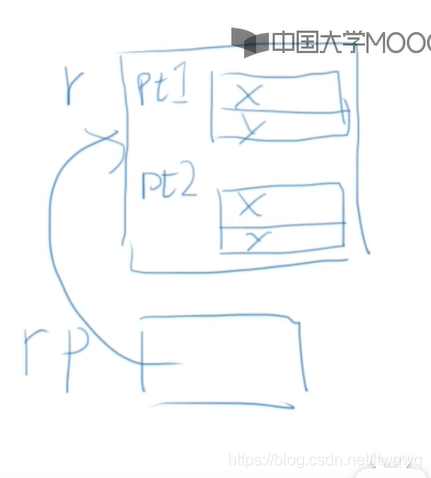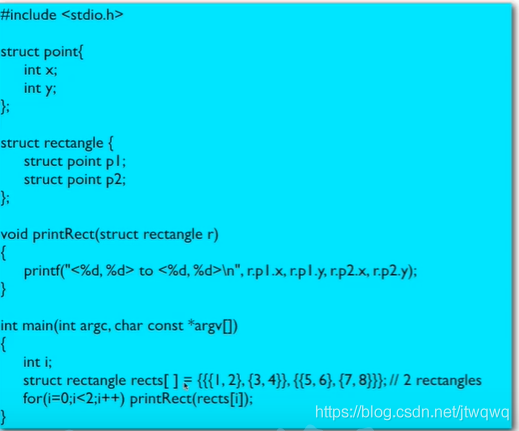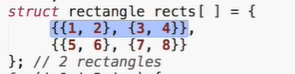（这里也能看出这么写会好看很多~）

## 类型定义

typedef struct ADate{int month;int day;int year;}Date;Date a={3,24,2021};

typedef *char String;//string是十个字符串的数组的类型。

## 联合

union，表面上与 struct 非常相似。

unit xxx{int a;char b;}xxx1,xxx2;xxx1.a=1;xxx2.b='c';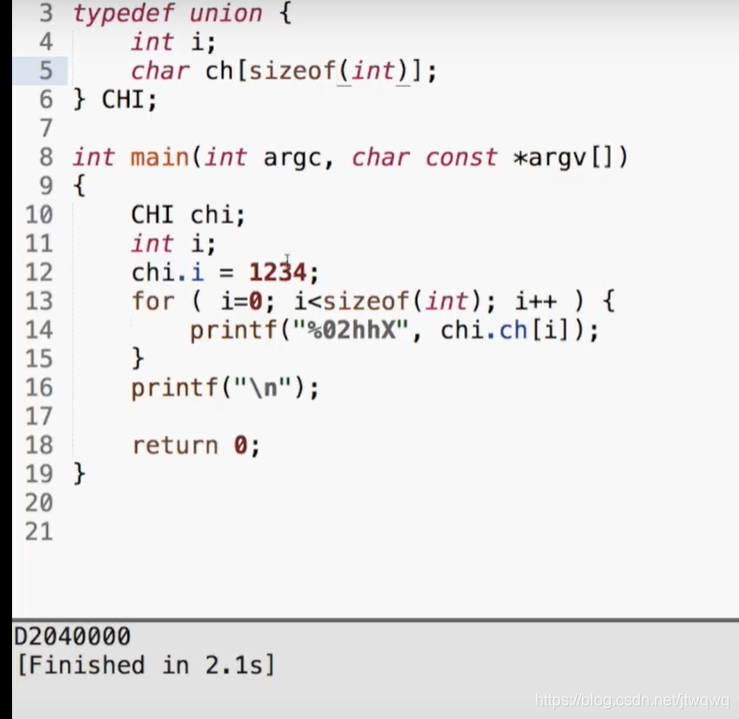# 12. 全局变量

## 全局变量：定义在函数之外的变量，全局的生存期和作用域

int gAll=12;int main(){  printf("in %s, gAll is %d",__func__,gAll);//__func__是输出当前函数名称  return 0;}

## 静态本地变量：能在函数结束后继续保持原值的本地变量

f(){  static int a=1;  a+=2;}

f()连续执行三次，不是每次都会 a=1，而是 1 3 3 5 5 7。静态本地变量实际是特殊的全局变量。他们位于相同的内存区域；（可以试着看下全局变量、本地变量、静态本地变量的地址 %p，静态本地变量和全局变量地址都是一样的）静态本地变量有全局内的生存期，但是只有函数内的局部作用域（static 在这里的意思是局部作用域，本地可访问。）

## 后记：返回指针的函数，使用全局变量的贴士

int *f();void g();int main(){  int *p=f();  printf("*p=%d",*p);  g();  printf("*p=%d",*p);  return 0;}int *f(){  int i=12;  return &i;}void g(){  int k=24;  printf("k=%d",k);  return 0;}

## 宏定义

#define PI 3.14159

gcc xxx.c --save-tempsls-l

tail xxx.c;tail xxx.i;

#define prt printf("123");\      printf("456");

__LINE__//行号__FILE__//文件名__DATE__//编译时日期__TIME__//编译时时间__STDC__

## 带参数的宏

printf("%d\n",cube(5));//输入tail 文件名，输出((5)*(5)*(5))

RADTODEG(x) (x*57.29578);RADTODEG(x) (x)*57.29578;RADTODEG(x) ((x)*57.29578);

# 13. 文件和位运算

## 多个源代码文件

main 中的代码太长，可以分出几个函数；而一个源代码文件(.c)太长可以分出很多.c 文件。如果我们直接把函数拿到另一个源代码文件里，编译很明显不会通过。我们需要新建一个项目。新建-项目，选择 console application 终端应用，把它保存到和两个目标源代码文件同一个文件夹里。新建之后会自带一个.c 文件，这个是假的，删掉即可。然后项目-添加，添加两个目标源代码文件，这样就可以编译并运行了。对于一个项目，Dev C++会把里面所有的文件编译并链接起来。其实 Dev C++是个比较特殊的(IDE)集成开发环境，绝大多数其他的 IDE 都需要你先建一个项目（哪怕只有一个源代码文件），然后才能进行其他操作。比如有的 IDE 是有编译和构建两个按钮，前者对单个源代码文件进行编译，后者对所有源代码文件链接。因为一个源代码文件是一个编译单元，编译器每次只对一个编译单元进行编译（形成.o 目标代码文件）。

## 头文件

#include"max.h"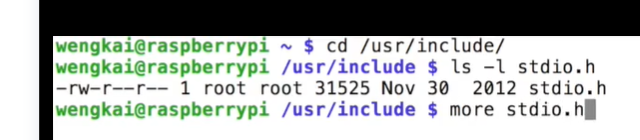## 声明

#ifndef __MAX_H__#define __MAX_H__//如果没有定义过max.h则定义max.h//中间是声明#endif

## 文件输入输出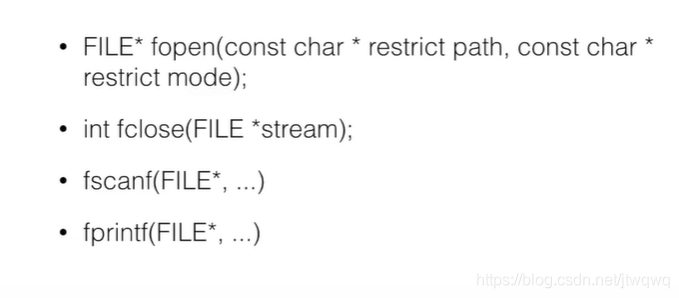FILE* fp=fopen("file","r");//file是文件名，r表示读if(fp){//如果没打开，会返回NULL  fscanf(fp,...);//读文件。省略号的东西和正常的scanf一样了。后面还可以printf  fclose(fp);}else{}//无法打开的反馈。如：输出 无法打开文件

## 二进制文件

1. 有配置（比如窗口大小、字体颜色）UNIX 用文本文件就能编辑，Windows 是用一个大文件：注册表编辑。

2. 数据：保存数据，如学生成绩。稍微大一些的数据都放数据库那了。

3. 媒体：如图片音频，这些不能是文本文件，只能是二进制文件。其实现在程序通过第三方库来读写文件，很少直接读写二进制文件了。对二进制的读写

4.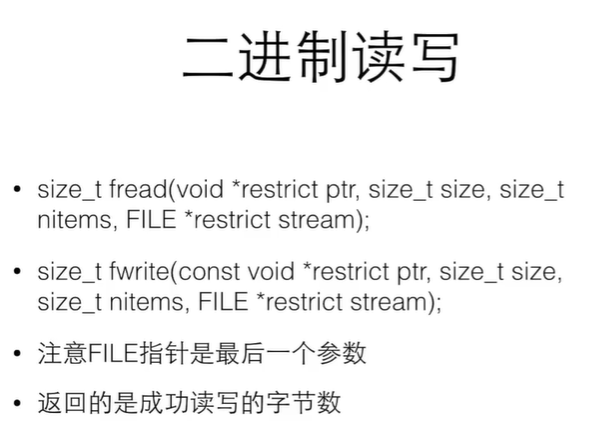5. 第一个参数是指针，要读写的内存；第二个参数是那块内存（一个结构）的大小；第三个参数是有几个这样的内存，第四个参数是文件指针。返回成功读写的字节数。因为二进制文件的读写一半是通过对一个结构变量的操作来进行的，所以 nitem 就是用来说明这次读写了几个结构变量。这里老师做了一个非常有意思的东西，建议去看看（我懒了）fwrite 可以把数据以二进制形式写到文件中，fread 类似。定位：找出现在处在文件中的位置，或跳到指定位置。

long ftell(FILE *stream);int fseek(FILE *stream, long offset, int whence);/*whence：SEEK_SET 从头开始SEEK_CUR 从当前位置开始SEEK_END 从末尾开始*/

## 按位运算

1. 让某一位或某些位=0，如 x & 0xFE（1111 1110） 会让最后一位变为 0

2. 取某个数中的某一段：如 x & 0xFF (在 32 位 int 中是 0000 0000 0000 0000 0000 0000 1111 1111）这样只会留下最后 8 位按位或应用：

3. 使得某一位/某几位变为 1： x | 0x01

4. 把两个数拼起来： 0x00FF | 0xFF00

## 移位运算

i<<j：i 中所有位向左移动 j 个位置，右边填入 0 所有小于 int 的类型，移位以 int 的方式做，结果是 int。往左移 1 位就等价于乘了 2.最多移动多少位取决于 int 的大小。x<<1 右移相当于/2。对于小于 int 的类型，移位以 int 的方式做，且结果是 int；对于 unsigned 类型，左边填 0 对于 signed 类型，左边填入原来的最高位（判断符号的 01），保持符号不变。而往左移动的时候是不管符号位的。移位的位数不要用负数，这是没有定义的行为！

## 位运算例子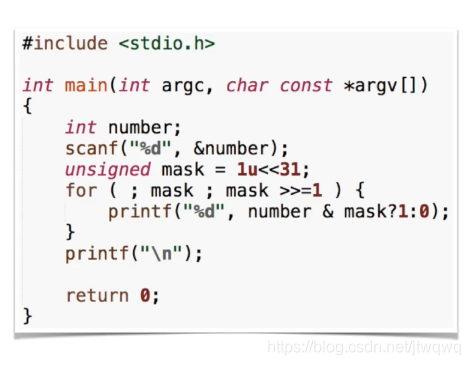unsigned 后面没有跟类型，则是默认为 unsigned int。mask 依次是 1000 00000100 00000010 0000...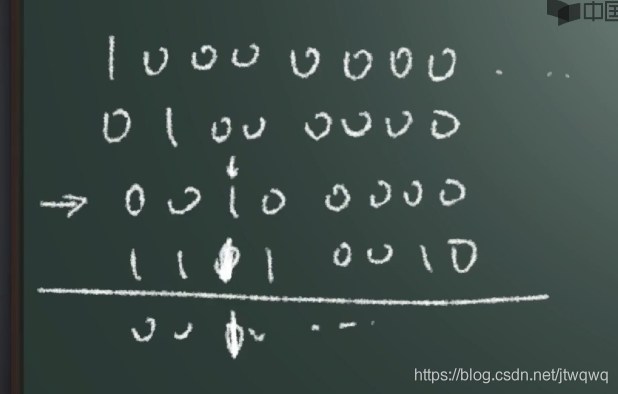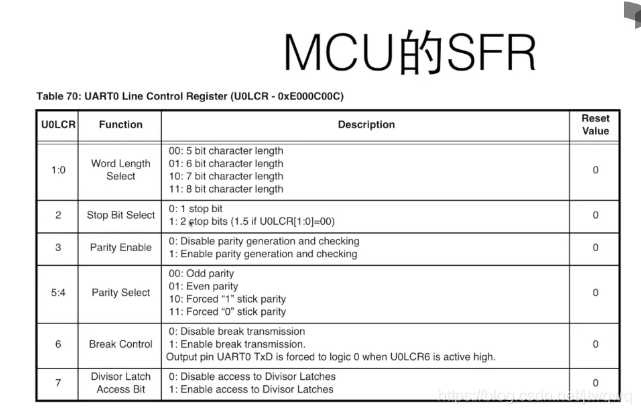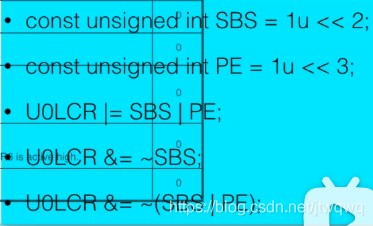## 位段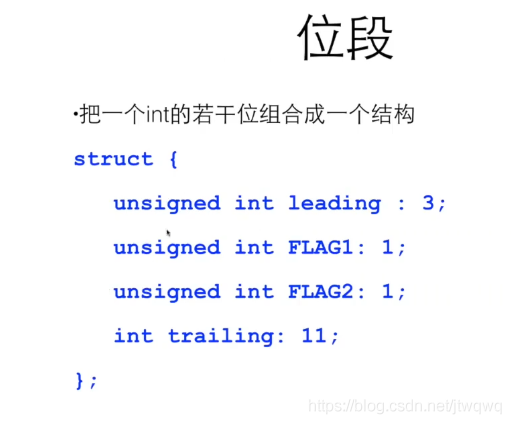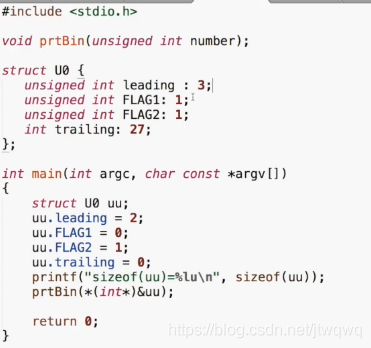prtBin 函数就是刚刚看过的输出二进制位。输出：sizeof(uu)=4 二进制输出后五位 10010 当我们把 uu.trailing=0 注释掉后，sizeof(uu)没变，但是二进制位输出变了。后五位仍然是 10010，前面没有赋初值（没有让 trailing=0），是乱七八糟的 0 和 1.当我们 int trailing=32，仍然让 uu.trailing=0，这时 sizeof(uu)=8（所有位数加起来超过了 32，所以用两个 int 来表达）二进制输出仍然只有后五位 10010.位段可以直接用位段的成员名称来访问，比移位、与、或还要方便。编译器会安排其中的位的排列，不具有可移植性（比如老师的例子是从最右边排起，可能自己试验时会从最左边排。）所需要的位超过一个 int 时会安排多个 int。总而言之，位段是操作和硬件相关的底层操作。

# 14. 可变数组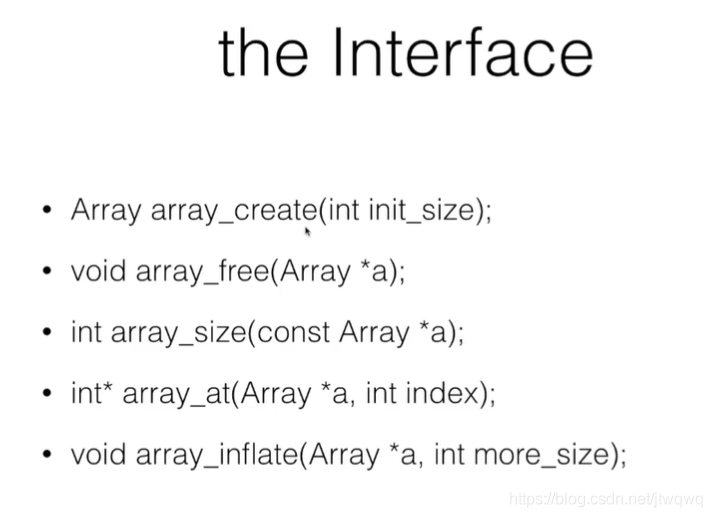create：创建数组 free：回收数组空间 size：告知单元数量 at：访问某个单元 inflate：长大结构 Array 包括*array 指针和 size 大小。

Array array_create(int init_size){  Array a;  a.size=init_size;  a.array=(int*)malloc(sizeof(int)*init_size);  return a;}

void array_free(Array *a){  free(a->array);  //保险起见，令a->size=0, a->array=NULL}

## 可变数组的数据访问

size：读入结构指针，直接 return a->size 即可。既然如此为啥不直接用 a.size 呢？第一种方法叫做封装，保护 a->size。今后随着版本升级、算法改进，可能 a->size 不能直接用了。at

int *array_at(Array *a, int index){  return &(a->array[index]);}

void array_get(const Array *a,int index){  return a->array[index];}void array_set(Array *a,int index,int value){  a->array[index]=value;}

## 可变数组自动增长

void array_inflate(Array *a, int more_size){  int *p=(int*)malloc(sizeof(int)*(a->size+more_size);  int i;  for(i=0;i<a->size;i++)p[i]==a->array[i];//把老空间里的东西放到新空间里去。  free(a->array);  a->array=p;  a->size+=more_size;}

while(number!=-1){  scanf("%d",&number);  *array_at(&a,cnt++)=number;}

## 可变数组的缺陷

1. 每次都要拷贝，很花时间

2. 每次都是在之前的内存结尾申请空间，不断往后排，所以最后其实我们不需要那么多内存空间，但我们的内存空间不够了。

3. 如果我们每次都只申请一块 block 大的内存，把他们链起来，不需要拷贝了节约时间，也可以充分利用给内存的每一块。

# 15. 链表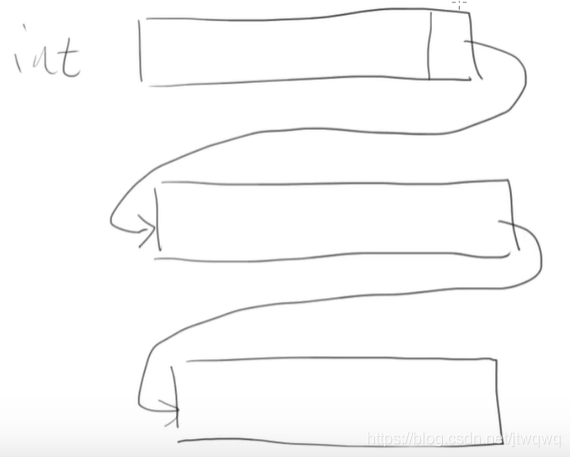typedef struct _node{  int value;  struct _node *next;}Node;

do{  scanf("%d",&number);  if(number!=-1)//add to linked-list}while(number!=-1);

Node *head=NULL;

Node *p=(Node*)malloc(sizeof(Node));p->value=number;p->next=NULL;//find the last, attachNode *last=head;if(last)//判断head是否为空{  while(last->next)last=last->next;  last->next=p;}else head=p;//即：p就是第一个结点

## 链表的函数

last=head;while(last->next)last=last->next;//找到结尾last结点last->next=p;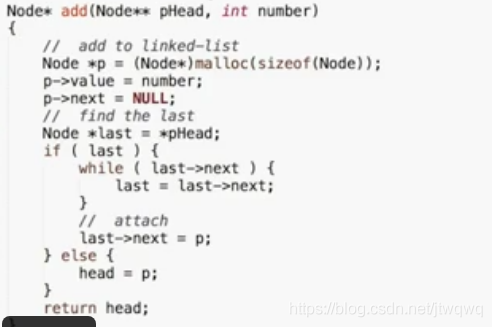## 链表的搜索

node *p;for(p=list.head;p;p=p->next)printf("%d\t",p->value);//遍历

for(p=list.head;p;p=p->next){  if(p->value==number)printf("找到了！");}

## 链表的删除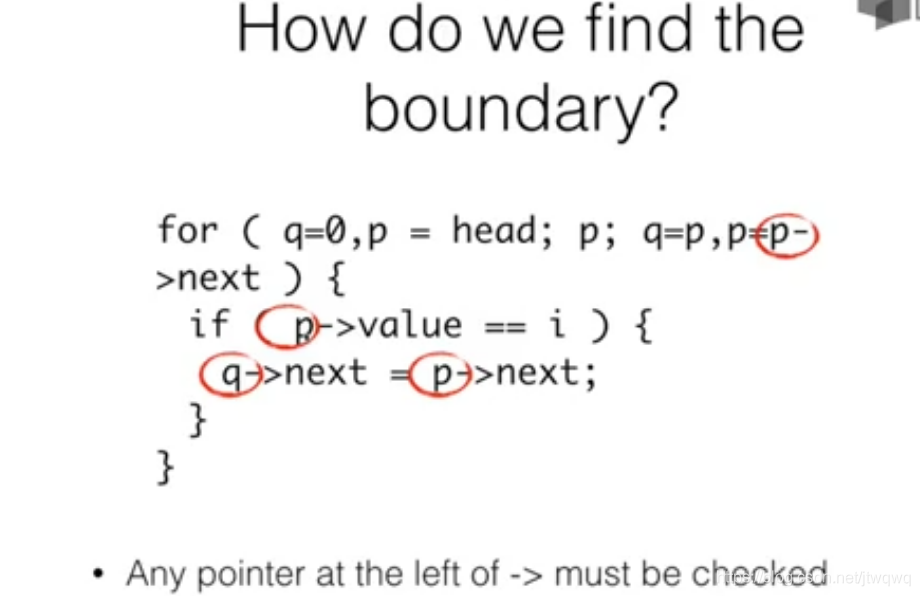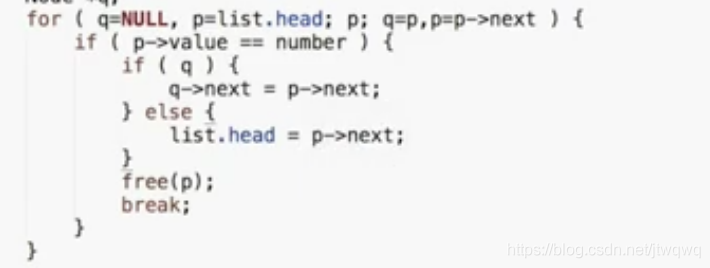## 链表的清除

for(p=head;p;p=q){  q=p->next;  free(p);}

# 完结## 评论